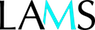# Sequence: Computation with Integers - EDUC261

Sequence Information
Sequence: Computation with Integers - EDUC261
Description:
Keywords: Mathematics: Computation with Integers
The LAMS sequence is evaluating Mathematics, specifically numbers and algebra with computation of integers. The learning outcomes the LAMS sequence shall be:
1.    Communicating and connecting the ideas of mathematics with specific terminology being used
2.    Appropriate techniques in correlation to mathematics helps solve problems
3.    Mathematical relationships are used to recognise and explain with reasoning
The LAMS sequence uses a visual aid to clarify the content listed below:
•    The content of this course shall cover the associative, communicative and distributive laws to help with mental and hand written retention of information
•    A non-calculator method that help with two or three numbers divided by a two digit number
•    Comparing the initial estimate with the answers that are obtained by methods that are written and checked by the calculator
•    A connection between division and multiplication, also when there is a remainder
(Example: 451/23 = 19 19/23 or 451 = 19 x 23 + 14)
•    Applying understanding through practicality of commutativity to help with mental comprehension
(Example: 3 + 9 = 9 + 3 = 12)
•    Applying understanding through practicality of associativity to help with mental comprehension
(Example: 3 + 8 + 2 = (3 + 8) + 2 = 13)
•    Determine that associativity only applies to multiplication of three or more and not for calculating division.
•    Practicality of distributive law to help comprehend a multiplication
(Example: multiply by 13; multiply number by ten then add onto a multiplication by 3)

Run time: 40 min

Delivery Mode: Visual

Outline of Activities: Multiple Choice, Examples, Short Answers, Explanations

Author view:

Audience: Middle school High school
Subjects: Mathematics
Language: English
LAMS Version: 2.5.0.
License:Attribution-Noncommercial-ShareAlike
Support Files: No files
Sharing with: Everyone in Public
Status: Active
Average Rating: Not rated yet!
Authored By:Lachlan Scott Wheeler